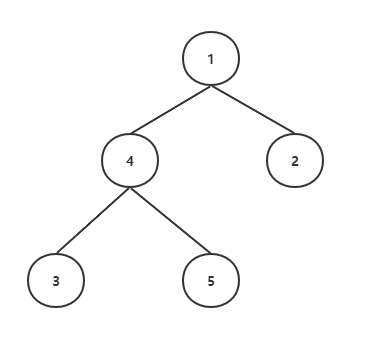## prufer序列

prufer序列是有标号无根树的一种编码，prufer序列和有标号无根树是一一对应的关系（可以相互转化）。

### 性质

• 度数为$d$的节点，只会在prufer序列中出现$d-1$次。
• $n$个节点的有标号无根树一共有$n^{n-2}$个。

prufer序列长度是$n-2$，每个位置都有$n$种可能。

• 有标号无根树森林的数量: $dp(n) = \sum_{i=0}^{n-1}\binom{n-1}{i} \ a(i+1) \ dp(n-1-i)$

• 对于给定度数的无根树，共有$\frac{(n-2)!}{\prod_{i=1}^{n}(d_i-1)!}$种情况。

### 有标号无根树转化为prufer序列### prufer序列转化为有标号无根树

1. 将prufer序列中最左边的点记为$u$。
2. 将在$S$中且不在prufer序列中且编号最小的点记为$v$。
3. 建立边$(u,v)$。
4. 删除prufer序列中最左边的点；从$S$中删除$v$。
5. 直到prufer序列为空，此时$S$中只剩下两个点，在这两点之间连一条边。

2020牛客多校7-I